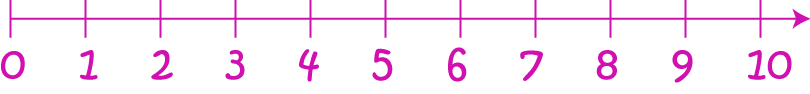# Definition of Natural Number

The natural numbers are usually considered to be the whole numbers, starting at $1$ and moving upwards like this: $1,2,3,4,\dots$

However, computer scientists (and some mathematicians) like to think of the natural numbers as starting with $0$. So they think the natural numbers are: $0,1,2,3,4,\dots$

Choose whichever one you like! It really doesn't matter where the natural numbers start, but it is important to remember that negative numbers and fractions are not natural numbers.### Description

The aim of this dictionary is to provide definitions to common mathematical terms. Students learn a new math skill every week at school, sometimes just before they start a new skill, if they want to look at what a specific term means, this is where this dictionary will become handy and a go-to guide for a student.

### Audience

Year 1 to Year 12 students

### Learning Objectives

Learn common math terms starting with letter N

Author: Subject Coach
Added on: 6th Feb 2018

You must be logged in as Student to ask a Question.

None just yet!